## Example Questions

### Example Question #1 : How To Find The Solution To An Inequality With Addition

Quantitative Comparison

Quantity A: 3x + 4y

Quantity B: 4x + 3y

Quantity B is greater.

The relationship cannot be determined from the information given.

The two quantities are equal.

Quantity A is greater.

The relationship cannot be determined from the information given.

Explanation:

The question does not give us any specifics about the variables x and y.

If we substitute the same numbers for x and y (say, x = 1 and y = 1), the two expressions are equal.

If we substitute different number in for x and y (say, x = 2 and y = 1), the two expressions are not equal.

If there are two possible outcomes, then we need more information to determine which quantity is greater. Don't be afraid to pick "The relationship cannot be determined from the information given" as an answer choice on the GRE!

### Example Question #221 : Algebra

Letbe an integer such that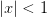.

Quantity A: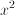Quantity B: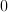Quantity A is greater.

Quantity A and Quantity B are equal.

The relationship cannot be determined from the information given.

Quantity B is greater.

Quantity A and Quantity B are equal.

Explanation:

The expressioncan be rewritten as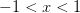.

The only integer that satisfies the inequality is 0.

Thus, Quantity A and Quantity B are equal.

### Example Question #225 : Algebra

Find all solutions of the inequality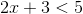.

All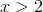.

All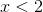.

All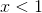..

All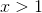.

All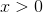.

All..

Explanation:

Start by subtracting 3 from each side of the inequality. That gives us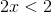. Divide both sides by 2 to get. Therefore every value forwhereis a solution to the original inequality.

### Example Question #21 : Inequalities

Find all solutions of the inequality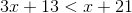.

All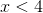.

All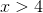.

All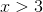.

All.

All.

All.

Explanation:

Start by subtracting 13 from each side. This gives us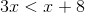. Then subtractfrom each side. This gives us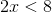. Divide both sides by 2 to get. Therefore all values ofwherewill satisfy the original inequality.

### Example Question #1 : How To Find The Solution To An Inequality With Addition

What values of x make the following statement true?

|x – 3| < 9

x < 12

–3 < x < 9

–12 < x < 6

6 < x < 12

–6 < x < 12

–6 < x < 12

Explanation:

Solve the inequality by adding 3 to both sides to get x < 12.  Since it is absolute value, x – 3 > –9 must also be solved by adding 3 to both sides so: x > –6 so combined.

### Example Question #11 : Inequalities

If –1 < w < 1, all of the following must also be greater than –1 and less than 1 EXCEPT for which choice?

|w|

w2

w/2

3w/2

|w|0.5

3w/2

Explanation:

3w/2 will become greater than 1 as soon as w is greater than two thirds. It will likewise become less than –1 as soon as w is less than negative two thirds. All the other options always return values between –1 and 1.

### Example Question #21 : Inequalities

Solve for.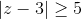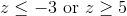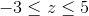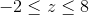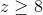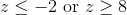Explanation:

Absolute value problems always have two sides: one positive and one negative.

First, take the problem as is and drop the absolute value signs for the positive side: z – 3 ≥ 5. When the original inequality is multiplied by –1 we get z – 3 ≤ –5.

Solve each inequality separately to get z ≤ –2 or z ≥ 8 (the inequality sign flips when multiplying or dividing by a negative number).

We can verify the solution by substituting in 0 for z to see if we get a true or false statement. Since –3 ≥ 5 is always false we know we want the two outside inequalities, rather than their intersection.

### Example Question #2 : How To Find The Solution To An Inequality With Addition

If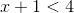and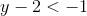, then which of the following could be the value of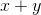?Explanation:

To solve this problem, add the two equations together: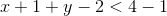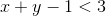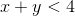The only answer choice that satisfies this equation is 0, because 0 is less than 4.

### Example Question #22 : Inequalities

What values ofmake the statement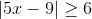true?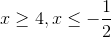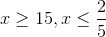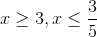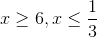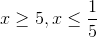Explanation:

First, solve the inequality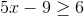: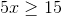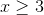Since we are dealing with absolute value,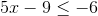must also be true; therefore: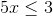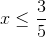Tired of practice problems?

Try live online GRE prep today.